Cherkashina A. S., Matsuga O. M., Atanova M. Y.

Oles Honchar Dnipropetrovsk National University

INFORMATION TECHNOLOGY OF DISTRIBUTIONS RESTORATION BASED ON ROBUST PROCEDURES

During the processing and analysis of sample data, anomalous observations which values are very different from the others require considerable attention. These observations can affect the results of statistical research significantly. To reduce their impact, it is advisable to apply robust methods of mathematical statistics. Such techniques are studied well, but their software implementation in automated data processing systems is still insufficient.

The paper sets the goal to develop software for solving the task of distribution restoration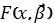, where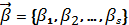– vector of distribution parameters, using robust methods.

Software’s kernel was created by a computational scheme of estimation the distribution parameters vector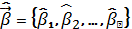based on robust procedure . The computational scheme can be presented as follows:

1.  Find an initial approximation of the estimate of the distribution parameters vector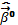using the least squares method, namely, by means of minimization of the residual variance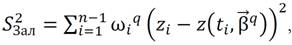(1)

where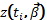– linearized distribution function;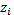– empirical value of linearized distribution function;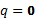– number of iteration;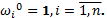2.  Set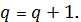3.  Using the current approximation of the estimate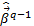and the chosen weight function, find the weight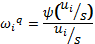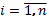,

where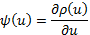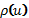– weight function;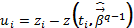q=1,2… – iteration number.

4.  Compute the following approximation of the estimate of the parameters vector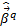by means of minimization of the functional (1), using the weights which were found in step 3.

5.  Check the condition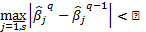. If this condition is satisfied, then we take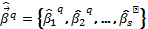for the desired evaluation of the distribution parameters vector. Otherwise, proceed to step 2.

The computational scheme was implemented to restoration of:

– normal, exponential, Weibull distributions;

– normal, exponential and Weibull spline-distributions with one node.

The following weight functions were used: Huber, Ramsey, Andrews, Tukey and Hampelya.

As a result, the software for distributions restoration by robust methods «RobustProcedurs» was created. Its testing was performed on the simulation data. Test results showed the adequacy of the computational scheme.

The created software «RobustProcedurs» based on the computational scheme forms the information technology of distributions restoration based on robust procedure. That ensures obtaining estimates which are relatively stable to statistical distribution of possible deviations from the theoretical.

The list of references:

1. Дрейпер Н. Прикладной регрессионный анализ: 3-е изд. / Н. Дрейпер, Г. Смит. – М.: Издательский дом «Вильямс», 2007. – 912 с.

2. Бабак В. П. Статистична обробка даних / В. П. Бабак, А. Я. Бі­ле­цький, О. П. Приставка, П. О. Приставка. – К.: МІВВЦ, 2001. – 388 с.